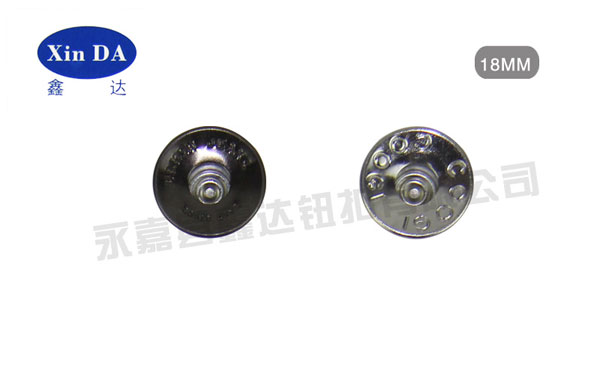# 简单了解：钮扣的大小分类：

a、按纽扣大小（即直径大小分）

b、纽扣的大小还有另外一种表示方式即L1L=0.625MM 1MM=1/25英寸 INCH) 表示方法单位:L,号(LIGNES)

12L=7.5 MM =5/16" 13L=8.0 MM =5/16" 14L=9.0 MM =11/32"

15L=9.5 MM =3/8" 16L=10.0 MM =13/32" 17L=10.5 MM =7/16"

18L=11.5 MM =15/32" 20L=12.5 MM =1/2" 22L=14.0 MM =9/16

24L=15.0 MM =5/8" 26L=16.0 MM =21/32" 28L=18.0 MM =23/32"

30L=19.0 MM =3/4" 32L=20.0 MM =13/16" 34L=21.0 MM =27/32"

36L=23.0 MM =7/8" 40L=25.0 MM =1" 44L=28.0 MM =1-3/32"

45L=30.0 MM =1-3/16" 54L=34.0 MM =1-5/16 60L=38.0 MM =1-1/2"

64L=40.0 MM =1-9/16"